## short communicationsJOURNAL OFSYNCHROTRONRADIATION
ISSN: 1600-5775
Volume 27| Part 3| May 2020| Pages 799-803

## Measurement of the horizontal beam emittance of undulator radiation by tandem-double-slit optical system

aGraduate School of Material Science, University of Hyogo, 3-2-1 Kouto, Kamigori, Ako, Hyogo 678-1297, Japan
*Correspondence e-mail: kagosima@sci.u-hyogo.ac.jp

(Received 2 September 2019; accepted 31 March 2020; online 15 April 2020)

A tandem-double-slit optical system was constructed to evaluate the practical beam emittance of undulator radiation. The optical system was a combination of an upstream slit (S1) and downstream slit (S2) aligned on the optical axis with an appropriate separation. The intensity distribution after the double slits, I(x1, x2), was measured by scanning S1 and S2 in the horizontal direction. Coordinates having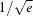intensity were extracted from I(x1, x2), whose contour provided the standard deviation ellipse in the x1x2 space. I(x1, x2) was converted to the corresponding distribution in the phase space, I(x1, x1′). The horizontal beam emittance was evaluated to be 3.1 nm rad, which was larger than the value of 2.4 nm rad estimated by using ray-tracing. It was found that the increase was mainly due to an increase in beam divergence rather than size.

### 1. Introduction

Third-generation synchrotron radiation facilities provide highly parallel X-ray beams with a low electron beam emittance of several nm rad; for instance, the natural electron beam emittance of SPring-8 is 2.4 nm rad (http://www.spring8.or.jp/en/about_us/whats_sp8/facilities/accelerators/storage_ring/). In storage rings, the electron beam shape in both position and angle spaces is a horizontally long ellipse; the electron beam sizes and divergences of SPring-8 are σx = 316 µm, σy = 4.9 µm, σx = 8.8 µrad and σy = 1.0 µrad, respectively. The large differences between the two directions are in some cases burdensome to optical systems requiring geometrical symmetry for incident beams. Particularly for experiments requiring spatial coherence, since a spatially coherent size at a large distance from the source is inversely proportional to the source size (Born & Wolf, 1986), the spatially coherent illumination area becomes a vertically long ellipse, which causes imbalance and asymmetry between the two directions in coherence. Upcoming and under-design synchrotron radiation facilities generally intend to improve the beam asymmetry, as well as the brightness.

A phase-space (position-angle space) description of a synchrotron X-ray beam is useful to visually understand the beam propagation properties (Matsushita & Kaminaga, 1980; Koch, 1983). The beam emittance is defined by an area of an ellipse divided by π in the phase space as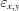=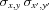(Koch, 1983). The diffraction-limited phase-space area in one direction is given by the condition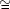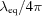, where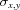(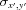) is theGaussian beam radius in position (angle) space and λeq is the shortest wavelength for which full spatial coherence is obtained (Attwood et al., 1985). Therefore, the measurement of beam properties in phase-space is a straightforward approach to evaluate the spatial coherence, and beam properties in phase-space should be assessed for experiments utilizing spatial coherence.

For measurements of beam emittance, several methods have been used (Chubar, 2000; Kube, 2007), namely visible-light imaging, X-ray imaging using X-ray optical elements or a pinhole camera (Ogata et al., 1989; Cai et al., 1995; Elleaume et al., 1995; Thomas et al., 2010; Kagoshima et al., 2017), and interference techniques (Mitsuhashi, 1997; Masaki & Takano, 2003). Intensity interferometry has also been studied using a high-resolution monochromator (Yabashi et al., 2001, 2004). Most of these methods are based on evaluation of the beam size, which directly relates to the spatial coherence properties. The presented method, termed tandem-double-slit optical system, is a modified version of the pinhole camera method. Arranging two slits with an appropriate distance in between, the beam position is measured using a front slit, and the beam angle is measured using the two slits, which function as an angular analyzer.

X-ray micro-imaging experiments have been performed at BL24XU of SPring-8 (Kagoshima et al., 2004, 2006). For scanning X-ray microscopy, a Fresnel zone plate (ZP) is used as the X-ray lens. In order to achieve the diffraction-limited focusing beam size, the ZP must be coherently illuminated. Coherent X-ray diffraction imaging (CXDI) has recently been attempted (Takayama et al., 2018) for achieving higher spatial resolution than X-ray microscopy using optical elements. The CXDI experiments also require coherent illumination. Thus, it is a key issue to quantitatively evaluate the coherent properties of incident beams for precise analyses of experimental data.

### 2. Beamline and optical system for measurement of beam emittance

Fig. 1shows an optical system constructed at the BL24XU beamline for measurement of intensity distribution in phase space of the incident beam. The photon energy of the fundamental harmonic peak of the undulator was tuned to 10 keV with the magnet gap of the undulator (insertion device gap; ID gap) of 11.3 mm. The polarization was horizontally linear. The effective beam sizes and divergences of the source Σx,y and Σx′,y are obtained as a convolution of the electron beam parameters (σx,y and σx′,y) and the natural photon beam parameters (σp = 2.65 µm and σp′ = 3.72 µrad for 10 keV at BL24XU), and had values of 317 µm, 5.57 µm, 9.52 µrad and 3.85 µrad, respectively. The X-ray beam was first collimated by a water-cooled four-quadrant front-end (FE) slit installed at 30 m from the source point U. The FE slit was opened to dimensions of 0.92 mm × 0.76 mm (height × width), which is the fully opened condition permitted for user operation. The beam was then monochromatized by a vertical dispersion SPring-8 standard liquid-nitro­gen-cooled double-crystal monochromator (Yamazaki et al., 2019) with silicon 111 symmetric reflection. Other beamline slits were sufficiently opened so that their geometrical effect on the downstream beam property was negligible. We adopted a tandem-double-slit optical system as an angular analyzer. The merit of this method is that it is much less complicated than the other methods and that the emittance can be obtained without knowledge of machine parameters such as the betatron function. In the experimental hutch B1, two narrow slits, S1 and S2 (20 µm aperture size), were mounted 5.565 m apart to construct the tandem-double-slit optical system. Since Σx is 8.8 µrad, the angular resolution of the angular analyzer must be of the same order or less than Σx. In order to fulfill this condition, we chose 20 µm aperture slits with a distance longer than 2.27 m (20 µm/2.27 m = 8.8 µrad). In order to maintain greater than a double margin, we took the distance of ∼5 m. The additional 0.565 m distance was because of other existing apparatus. A PIN photodiode was used as an intensity detector. A beam monitor was installed at the downstream end.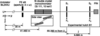Figure 1 Optical system (top view) for the measurement of intensity distribution in phase space of the incident beam. (Constructed at BL24XU of SPring-8).

### 3. Beam patterns and phase space intensity distribution

It is well known that far-field beam patterns of undulator radiation strongly depend on the ID gap. Fig. 2shows the intensity and the beam pattern changes, as the ID gap was varied, for the fundamental harmonic intensity peak. At the highest intensity, point B, the beam pattern shows a vertical splitting. At the larger gap, point C, the vertical splitting grows. At the smaller gap, point A, the splitting vanishes. We chose point A to measure the intensity distribution in phase space.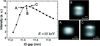Figure 2 Intensity changes with variation of the ID gap (left). The beam pattern also changes with the ID gap (right).

Intensity distribution was measured by scanning S1 and S2 with a step size of 10 µm. The acquisition time is 0.1 s. Let the horizontal positions of the two slits be x1 and x2, and the measured intensity distribution be I(x1, x2). Fig. 3(a)shows I(x1, x2). A slope of 45° means that a beam is parallel; thus, the slightly larger slope shows that the beam was gradually expanding. The black thick line is a contour having the intensity of, which corresponds to the standard deviation ellipse usually used in beam description.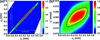Figure 3 (a) Measured intensity distribution I(x1, x2). The black thick line is a contour having intensity of(= 0.6065). The horizontalintensity widths were 0.94 mm at S1 and 1.04 mm at S2. (b) Horizontal phase-space intensity distribution I(x1, x1′) calculated by equation (1). The black thick line is the standard deviation ellipse in the phase space.

I(x1, x2) was converted to the corresponding intensity distribution in the phase space, I(x1, x1′), at S1 by using the next free space beam transfer matrix (Matsushita & Kaminaga, 1980; Ogata et al., 1989),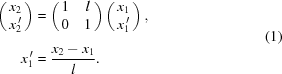Fig. 3(b)shows the thus derived I(x1, x1′). The black thick line is the standard deviation ellipse in the phase space. The beam emittance is defined by the area of an ellipse divided by π in phase space (Koch, 1983; Attwood et al., 1985). Since I(x1, x1′) is a convolution of the net phase-space distribution of the beam, as is required, with the Fraunhofer diffraction at S1 illuminated by a plane wave; thus, the Fraunhofer diffraction should be deconvoluted. The Fraunhofer diffraction pattern as a function of diffraction angle θ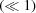is given as I(θ) ∝ sinc2(πaθ/λ), where λ is the wavelength and a is the slit aperture. The Fraunhofer diffraction represented in the phase space is shown in Fig. 4(a), where a = 20 µm and x1′ = θ. The intensity color scale is plotted logarithmically to emphasize the subsidiary structures of the sinc2 function. The diffraction angle giving the first minimum is given by x1′ = λ/a = 6.2 µrad. Thus, the emittance after a slit for the Fraunhofer diffraction, SF, is given by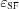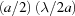=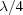, which corresponds to the diffraction-limited phase-space volume measured by the half beam width and divergence (Attwood et al., 1985). Furthermore, when scanning S2, its finite width increases the effect on the x′ space. The full angular width of S2 is a/l = 3.6 µrad. Since the two values are comparable, the effect of S2 also needs to be eliminated. Fig. 4(b)shows the convoluted Fraunhofer diffraction [Fig. 4(a)] with S2 in x′ space, where the subsidiary structures are no longer conspicuous.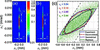Figure 4 (a) Fraunhofer diffraction of S1 represented in phase space. (b) Convoluted Fraunhofer diffraction with S2 in x′ space. (c) Blue: measured phase-space ellipse [identical to the black thick line in Fig. 3(b)]; red: deconvoluted phase space ellipse; purple: geometrically propagated source ellipse partly cut by the FE slit; green: ray-tracing; black dashed: geometrically propagated FE slit. The leftmost x1′ scale is common to (a), (b) and (c). The intensity color scale is common to (a) and (b).

The deconvolution was performed by using an unsupervised Wiener–Hunt deconvolution algorithm (Orieux et al., 2010) implemented in scikit-image in Python (van der Walt et al., 2014; see also https://scikit-image.org/docs/dev/api/skimage.restoration.html#skimage.restoration.unsupervised_wiener). Here, the impulse response (psf) is the convoluted Fraunhofer diffraction with S2 [Fig. 4(b)], and the degraded image is the experimentally measured phase-space intensity distribution I(x1, x1′) [Fig. 3(b)]. Fig. 4(c)shows the thus processed phase space ellipses, where the blue line is the experimental ellipse and the red line is the deconvoluted phase space ellipse, accompanied by a purple line as a geometrically propagated source ellipse partly cut by the FE slit, green dots, and a line as a ray-tracing result taking the dynamical diffraction of perfect crystal into account, and a pair of black dashed lines as a geometrically propagated FE slit, respectively. We used a ray-tracing calculation program developed by Muramatsu et al. (1988) for beam propagation and optical element functions. We appended the dynamical diffraction effect of a perfect crystal to the program. For the emission of rays from the undulator, we used SHADOW3 (Sanchez del Rio et al., 2011). The two-dimensional kernel density map of the ray-tracing result was calculated, and itscontour is shown as the green line. The edge of the distribution of green dots corresponds well to the geometrically propagated FE slit (black dashed lines), and the geometrically propagated source ellipse, partly cut by the FE slit (purple line) and thecontour of the ray-tracing result (green line), are almost the same, except for the side end region. To avoid an excessively high dot density, the displayed number of rays (green dots) was properly thinned out. The areas of the ellipses divided by π are shown in Fig. 4(c). The emittance was evaluated to be 3.1 nm rad (red ellipse), which was larger than the value of 2.4 nm rad estimated by using ray-tracing (green line). It was found that the increase was mainly due to an increase in beam divergence rather than size. The reason is not identified at present.

### 4. Discussions

In order to fully understand the beam properties of a beam incident into the experimental station, the intensity distribution as a function of position (x, y), angle (x′, y′) and wavelength (λ) should be measured. The position-angle space is a phase space and the angle-wavelength space corresponds to the DuMond diagram. As far as the authors know, both the phase space and DuMond diagram have never been simultaneously measured. Our major motivation is to simultaneously obtain the beam properties in both spaces. To do this, conserving position and angle of an incident beam, the beam should be incident on a wavelength analyzer crystal having sufficiently higher wavelength resolution than the beamline monochromator. Since the other methods may not be directly applicable for this purpose, we adopted the pinhole camera method. The pinhole camera can be easily equipped with a wavelength analyzer crystal in its end. The presented experiment is an initial step for the measurement of five-dimension beam properties in (x, y; x′, y′; λ) space.

One of the key issues is whether the presented method is applicable to the multi-bend achromat lattice rings with a horizontal emittance ranging from 100 to 10 pm rad. If the source size becomes small, the effect of Fraunhofer diffraction by S1 becomes dominant in intensity distribution. In order to remove the effect, we introduced a method deconvoluting the Fraunhofer diffraction of S1 in phase space as described in Section 3. In order to obtain high-resolution intensity distribution in phase space, the aperture size of the slits should be smaller. On the other hand, the smaller aperture size causes lower intensity, which leads lower signal-to-noise ratio. Therefore, the limitation of the presented method depends on how accurately the Fraunhofer diffraction can be deconvoluted from the measured intensity distribution. In the same way as usual experiments, the limitation may be determined by the noise level of the measurements. If the noise is zero, the emittance is correctly calculated. In order to study the limitation caused by noise, we additionally made simple simulations. Assuming the horizontal beam size and divergence (σx, σx) to be (27.3 µm, 4.96 µrad) as designed for SPring-8 II (SPring-8-II, 2014), the effective beam size and divergence (Σx, Σx) are calculated to be (27.5 µm, 7.02 µrad) for the first-harmonic photon energy of 5625 eV, which corresponds to the same gap of the insertion devise. Therefore, the horizontal effective beam emittance is ΣxΣx = 193 pm rad. We set simulation conditions as follows: the width of S1 and S2 is 10 µm, l is 10 m and the scanning step of S1 and S2 is 2.5 µm. The source intensity distribution in phase space is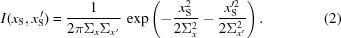Using equation (1), I(xS, xS′) was converted to the intensity distribution at S1, I(x1, x1′). Convolution of the Fraunhofer diffraction by S1 with angular acceptance of S2 was calculated in phase space as the impulse response (psf). Next, I(x1, x1′) was convoluted with psf, and the noiseless intensity distribution INL(x1, x1′) was prepared. Gaussian noise with a mean of zero and several standard deviations σ was added to INL(x1, x1′) using image processing program ImageJ (Schneider et al., 2012). Finally, the same deconvolution was executed as carried out in Fig. 4(c). Fig. 5shows the results for σ of 0–0.02%. A σ value of 0.02% means here that σ = 0.0002 × the maximum value of INL(x1, x1′). The emittance change is roughly constant with a mean value of ∼220 pm rad. In the cases of 0.02%, the main ellipse started to collapse. For Fig. 3(a), σ in the background level divided by the maximum value can be estimated to be roughly 0.02% with an acquisition time of 0.1 s. Therefore, if intensity is measured with a sufficiently low noise level, several hundred pm rad can be estimated by the presented method.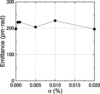Figure 5 Calculated emittance changes for several σ of Gaussian noise added to noiseless image.

In summary, a tandem-double-slit optical system was constructed to evaluate the practical beam emittance of undulator radiation incident on experimental stations. The horizontal phase-space intensity distribution was derived. The net beam emittance was extracted by deconvoluting the Fraunhofer diffraction by the slit in phase space. The experimentally evaluated beam emittance was larger than the value estimated by using ray-tracing. It was found that the increase was mainly due to an increase in beam divergence rather than size. It was confirmed that this tandem-double-slit optical system is effective for facile evaluation of beam properties in synchrotron radiation beamlines.

### Acknowledgements

The synchrotron radiation experiments were performed at BL24XU of SPring-8 with the approval of the Japan Synchrotron Radiation Research Institute (JASRI) (Proposal Nos. 2018A3200 and 2018B3200). We would like to thank Editage (http://www.editage.jp) for English language editing.

### ReferencesJOURNAL OFSYNCHROTRONRADIATION